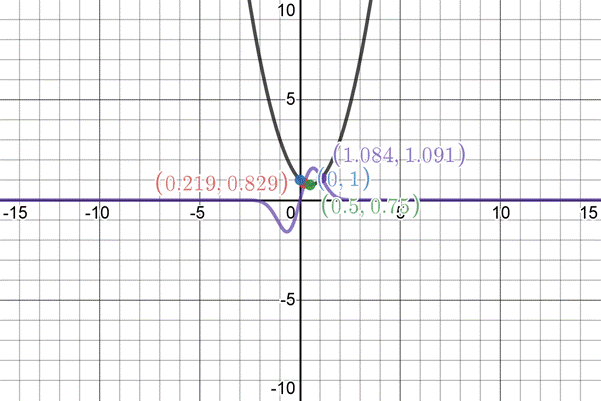# The roots of the equation, 3 e − x 2 sin x = x 2 − x + 1 .### Single Variable Calculus: Concepts...

4th Edition
James Stewart
Publisher: Cengage Learning
ISBN: 9781337687805### Single Variable Calculus: Concepts...

4th Edition
James Stewart
Publisher: Cengage Learning
ISBN: 9781337687805

#### Solutions

Chapter 4.7, Problem 21E
To determine

## To calculate: The roots of the equation,  3e−x2sinx=x2−x+1 .

Expert Solution

The roots are

x2=0.10211882

x3=0.24339599 .

### Explanation of Solution

Given information:

The equation is given as:

3ex2sinx=x2x+1 .

Formula used:

Newton’s Method:

We seek a solution of f(x)=0 , starting from an initial estimate x=x1 .

For x=xn , compute the next approximation xn+1 by

xn+1=xnf(xn)f'(xn) and so on.

Calculation:

Consider the equation,

3ex2sinx=x2x+1

The initial approximation x1 can be guessed by sketching both the graphs

y=3ex2sinx and y=x2x+1 .Both the graph intersect at four points whose x-coordinate can be seen in graph.

Let x1=0.5 where

f(x)=3ex2sinxx2+x1f'(x)=3ex2[cosx2xsinx]2x+1

Now, at n=1

x2=x1f(x1)f'(x1)

x2=x1{3ex12sinx1x12+x113ex12[cosx12x1sinx1]2x1+1}

x2=(0.5)(3e(0.5)2sin(0.5)(0.5)2+0.513e(0.5)2[cos(0.5)2(0.5)sin(0.5)]2(0.5)+1)x2=(0.5)(1.1201309550.25+0.510.9302550041+1)x2=(0.5)(0.3701309550.930255004)

x2=0.5(0.397881176)x2=0.50.397881176x2=0.102118824

The second approximation is x2=0.10211882 .

Let n=2 ,

x3=x2f(x2)f'(x2)

x3=x2{3ex22sinx2x22+x213ex22[cosx22x2sinx2]2x2+1}

x3=(0.10211882)(3e(0.10211882)2sin(0.10211882)(0.10211882)2+0.1021188213e(0.10211882)2[cos(0.10211882)2(0.10211882)sin(0.10211882)]2(0.10211882)+1)x3=(0.10211882)(0.3387049920.010428253+0.1021188213.2360597470.20423764+1)x3=(0.10211882)(0.5696044414.031822107)

x3=0.10211882(0.141277175)x3=0.10211882+0.141277175x3=0.243395995

The third approximation with six decimal places is x3=0.24339599 .

Therefore, the roots with eight decimal places are :-

x2=0.10211882

x3=0.24339599

### Have a homework question?

Subscribe to bartleby learn! Ask subject matter experts 30 homework questions each month. Plus, you’ll have access to millions of step-by-step textbook answers!